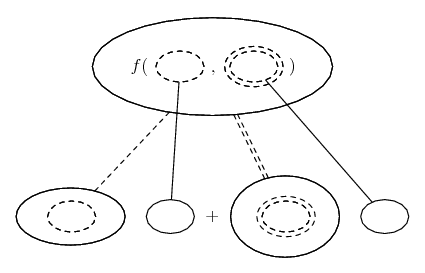# Thread: Help with multivariable calculus problem

1. ## Help with multivariable calculus problem

Hi guys,

I've got a question set for uni and I'm having trouble with it. There are two questions I'm having trouble with. Here they are.

Q1: apply the definition of the limit to prove the following limit exists
lim x^3 + 4x^2y
(x,y)->(0,0) x^2+2y^2

Q3: z(u,v)=f(x(u,v),y(u,v))
x(u,v)=u^2-v^2 y(u,v)=2uv

show that fxx + fyy = (fuu + fvv)/ (4(u^2+v^2))

fxx being the partial, double derivative with respect to x, fyy with respect to y etc.

For Q1, I'm not very good with limits. Apparently we need to do something called a epsilon delta equation and when I looked that up, I got really confused. Even when I try evaluating the limit along different paths, e.g. the x axis, y axis, y=mx etc. I get different values so I'm really confused. You obviously can't evaluate the limit at (0,0) because the function is discontinuous but I'm just not sure what to do.

When I evaluate x->0 I get limit=0
When I evaluate y->0 I get limit=x (and I thought if you evaluated x->0 further you'd still get 0 but I'm not sure if this is right)

is the limit 0? Help meAlso, how would I go about the epsilon delta thingy?

As for Q3. I took the hint and worked with the right hand side. I expected to get a neat little expression for the double derivatives with respect to u and v in terms of the double derivatives with respect to x and y but it just didn't happen too well. Here is a screen capture of what I tried doing and what I ended up with. Maybe I made an arithmetic error but I've tried this question dozens of times.I really need help. All and any is welcome and appreciated.

Thank you.

2. ## Re: Help with multivariable calculus problem

Oops. For Q1 it meant to look like this....

Q1: apply the definition of the limit to prove the following limit exists
lim
(x,y)->(0,0)

x^3 + 4x^2y
x^2+2y^2

3. ## Re: Help with multivariable calculus problem

Just in case a picture helps with Q3...... where (key in spoiler) ...

Spoiler:is the chain rule for two inner functions, i.e...

$\displaystyle \frac{d}{du}\ f(x(u), y(u)) = \frac{\partial f}{\partial x} \frac{dx}{du} + \frac{\partial f}{\partial y} \frac{dy}{du}$

As with...... the ordinary chain rule, straight continuous lines differentiate downwards (integrate up) with respect to, say, u, and the straight dashed line similarly but with respect to the (corresponding) dashed balloon expression which is (one of) the inner function(s) of the composite expression. (In this case x or y.)

To differentiate a second time we have to embed the above in the legs-uncrossed version of... the product rule, where, again, straight continuous lines are differentiating downwards with respect to u or v.

Add the bottom rows and simplify.

_________________________________________

Don't integrate - balloontegrate!

Balloon Calculus; standard integrals, derivatives and methods

Balloon Calculus Drawing with LaTeX and Asymptote!

4. ## Re: Help with multivariable calculus problem

Ohhhh I see now. My problem was I wasn't using the product rule for the second derivatives because I assumed that you could take all the u and v terms out as a constant. Thank you so much for your help!!!! By the way, you made a slight error (not that it matters) but for your fvv, you have 2(fxy.-2v + fyy.2v). The two in front of the brackets should be 2u.

I'm still stumped for question 1. I'm not very good with limits :S

### calculus

Click on a term to search for related topics.

#### Search Tags

calculus, multivariable, problem